# Reservoir

6 m long reservoir has a diameter of 2.2 m. What is its surface area in square meters?

Correct result:

S =  49.0717 m2

#### Solution: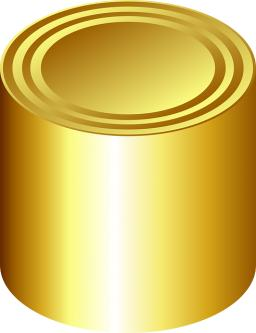We would be pleased if you find an error in the word problem, spelling mistakes, or inaccuracies and send it to us. Thank you!#### You need to know the following knowledge to solve this word math problem:

We encourage you to watch this tutorial video on this math problem:

## Next similar math problems:

• Water reservoir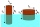What is the weight of a metal reservoir - cylinder with a diameter of 2 m and a length 8 m, if 1 m2 of sheet metal weighs 100 kg?
• Rotary cylinder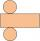The rotating cylinder has a surface area 69.08 cm2. The area of the shell is 62.8 cm 2. What is the diameter of the cylinder?
• Posters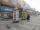Column with posters in the form of a cylinder is 2 m high and its diameter is 1.7 m. What is the content area for which it is possible to stick posters?
• Tin with oilTin with oil has the shape of a rotating cylinder whose height is equal to the diameter of its base. Canned surface is 1884 cm2. Calculate how many liters of oil is in the tin.
• Pine woodFrom a trunk of pine 6m long and 35 cm in diameter with a carved beam with a cross-section in the shape of a square so that the square had the greatest content area. Calculate the length of the sides of a square. Calculate the volume in cubic meters of lu
• The cylindrical containerThe container has a cylindrical shape the base diameter 0.8 meters has a content area of the base is equal to the content area of the shell. How many full liters of water can be poured maximally into the container?
• The hollow cylinderThe hollow cylinder has a height of 70 cm, an outer diameter of 180 cm and an inner diameter of 120 cm. What is the surface of the body, including the area inside the cavity?
• The aquariumThe aquarium has a capacity of 18 liters. What is its height when the square bottom is 8 2/3 cm long?
• Fountain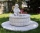The stone fountain, which has the shape of a cylinder with a diameter of 3 m, is 70 cm deep. How many m2 of stone is wetted with water?
• RollerCylinder shell has the same content as one of its base. Cylinder height is 15 dm. What is the radius of the base of the cylinder?
• Half-sphere roofThe roof above the castle tower has the shape of a 12.8 m diameter half-sphere. What is the cost of this roof, if the cost of 1 square meter is 12 euros and 40 cents?
• CD disc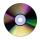A compact disc has a diameter of 11.8 cm. What is the surface area of the disc in square centimeters?
• Roller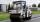Roller has a diameter of 0.96 m and a width 169 cm. How many m2 of road level when he turns 42-times?
• Cylindrical tank9.6 hl of water is poured into a cylindrical tank with a bottom diameter of 1.2 m. What height in centimeters does the water reach?
• Concrete pipe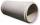Concrete pipe is cylindrical with an inner diameter 110 cm and outer 120 cm. Calculate the surface of the concrete pipe, if it is 9 m long.
• Gasoline tank cylindrical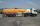What is the inner diameter of the tank, which is 8 m long and contains 40 cubic cubic meters of gasoline?
• GreenhouseGarden plastic greenhouse is shaped half cylinder with a diameter of 6 m and base length 20 m. At least how many m2 of plastic is need to its cover?